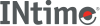﻿ arc4randomINtime SDK Help
arc4random

The arc4 random number generator.

```#include <stdlib.h>

uint32_t
arc4random(void);

void
arc4random_buf(void *buf, size_t nbytes);

uint32_t
arc4random_uniform(uint32_t upper_bound);

void
arc4random_stir(void);

void
```

#### Description

The `arc4random()` function uses the key stream generator employed by the arc4 cipher, which uses 8*8 8 bit S-Boxes. The S-Boxes can be in about (2**1700) states. The `arc4random()` function returns pseudo-random numbers in the range of 0 to (2**32)-1, and therefore has twice the range of rand.

`arc4random_buf()` function fills the region buf of length nbytes with ARC4-derived random data.

`arc4random_uniform()` will return a uniformly distributed random number less than upper_bound. `arc4random_uniform()` is recommended over constructions like '`arc4random() % upper_bound`' as it avoids "modulo bias" when the upper bound is not a power of two.

The `arc4random_stir()` function reads data from available sources of "Operating System entropy" and uses it to permute the S-Boxes via `arc4random_addrandom()`.

There is no need to call `arc4random_stir()` before using `arc4random()` functions family, since they automatically initialize themselves.

#### Example

The following produces a drop-in replacement for the traditional `rand()` and `random()` functions using `arc4random()`:
#define foo4random() (arc4random() % ((unsigned)RAND_MAX + 1))

#### Requirements

Versions Defined in Include Link to
INtime 6.0 intime/rt/include/stdlib.h stdlib.h clib.lib